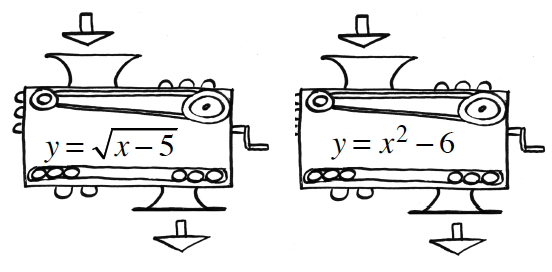### Home > CC4 > Chapter 1 > Lesson 1.1.1 > Problem1-6

1-6.Angelica is working with function machines. She has the two machines shown at right. She wants to put them in order so that the output of the first machine becomes the input of the second. Her initial input is $6$. Homework Help ✎

1. In what order must she put the machines to get a final output of $5$?

Substitute $6$ for $x$ in the first function.

${y=\sqrt{6-5}}$

$y = 1$

Let the output from the first equation become the input, $x$, in the second equation.

$y = 1^2 − 6$
$y = −5$
Incorrect. Try the other order.

Substitute $6$ for $x$ in the 2nd equation.

$y = 6^2 − 6$
$y = 30$

Let the output from the second equation become the input, x, in the fist equation.

${y = \sqrt{30 - 5}}$

$y = 5$
Correct!

2. Is it possible for her to find an initial input that will get a final output of $-5$? If so, show how she could do that. If not, explain why not.

• Which equation cannot have a negative output? What input would you need from the other equation to get $-5$ as an output? See part (a) for more help.